ConicBundle
ConicBundle::Bigmatrix Class Reference

AffineMatrixFunction needs to compute the maximum eigenvalue of an affine matrix function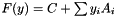. This class prepares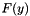in useful form for iterative eigenvalue solvers. More...

#include <Bigmatrix.hxx>

Inheritance diagram for ConicBundle::Bigmatrix: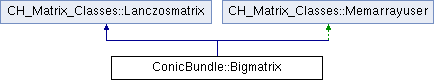## Public Member Functions

void clear ()
clear all data

CH_Matrix_Classes::Integer get_nmult () const
return number of matrix vector products

void reset_nmult ()
reset the counter of matrix vector products to zero

int init (const CH_Matrix_Classes::Matrix &yi, CH_Matrix_Classes::Integer indim, const CoeffmatPointer C, const SparseCoeffmatVector *Ai, const bool dense=false)
compute a good representation of(if dense==true directly a dense one)

void set_tol (CH_Matrix_Classes::Real t)
sets the tolerance for considering computed values as zeros

int get_dense () const
return true if a dense representation was computed

virtual CH_Matrix_Classes::Integer lanczosdim () const
the order of the matrix as required by CH_Matrix_Classes::Lanczosmatrix

virtual CH_Matrix_Classes::Integer lanczosflops () const
the estimated flop count of a matrix vector multiplication as required by CH_Matrix_Classes::Lanczosmatrix

virtual int lanczosmult (const CH_Matrix_Classes::Matrix &A, CH_Matrix_Classes::Matrix &B) const
computes B = bigMatrix * A (A and B must not refer to the same memory) as required by CH_Matrix_Classes::Lanczosmatrix

int make_symmatrix (CH_Matrix_Classes::Symmatrix &S) const
write a dense version of the current matrix to S

const CH_Matrix_Classes::Symmatrixget_symrep () const
compute the dense version locally and return a reference to it

## Private Types

typedef std::map< CH_Matrix_Classes::Integer, CH_Matrix_Classes::RealIndexhash
for collecting the indices of sparse data this seems useful

## Private Attributes

CH_Matrix_Classes::Real tol
tolerance for considering an element to be zero

CH_Matrix_Classes::Integer dim
size of the matrix

CH_Matrix_Classes::Integer nz
total number of nonzeros (except diagonal)

CH_Matrix_Classes::Indexmatrix colnz
nonzeros of each column

std::vector< CH_Matrix_Classes::Indexmatrixrowind
nonzero row indices in each column

std::vector< CH_Matrix_Classes::Matrixrowval
corresponding nonzero row values

CH_Matrix_Classes::Matrix di
diagonal elements

std::vector< Indexhashrowhash
for finding nonzero indices

std::vector< CoeffmatPointermcp
pointers to dense matrices

CH_Matrix_Classes::Matrix mcv
multiplier values for the dense matrices

CH_Matrix_Classes::Integer nmult
counts the number of Mat*vec operations

bool use_dense
usually false, set to true if matrix is sufficiently dense

CH_Matrix_Classes::Symmatrix symrep
if use_dense is true, the matrix is stored here

bool symrep_init
flag whether symrep is computed already

CH_Matrix_Classes::Matrix At
auxilliary variable used in matrix vector products

CH_Matrix_Classes::Matrix Bt
auxilliary variable used in matrix vector products

CH_Matrix_Classes::Indexmatrix collecti
for collecting the sparse part

CH_Matrix_Classes::Indexmatrix collectj
for collecting the sparse part

CH_Matrix_Classes::Matrix collectval
for collecting the sparse part

## Friends

std::ostream & operator<< (std::ostream &out, const Bigmatrix &)
output informationProtected Member Functions inherited from CH_Matrix_Classes::Memarrayuser
Memarrayuser ()
if memarray is NULL, then a new Memarray is generated. In any case the number of users of the Memarray is incremented

virtual ~Memarrayuser ()
the number of users is decremented and the Memarray memory manager is destructed, if the number is zero.Static Protected Attributes inherited from CH_Matrix_Classes::Memarrayuser
static Memarraymemarray
pointer to common memory manager for all Memarrayusers, instantiated in memarray.cxx

## Detailed Description

AffineMatrixFunction needs to compute the maximum eigenvalue of an affine matrix function. This class preparesin useful form for iterative eigenvalue solvers.

In AffineMatrixFunction the matrices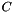and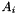are of type Coeffmat, the information provided by these matrices is used to decide which parts should be represented in a sparse form and which parts in a dense form. The intention is that in the end matrix vector products can be computed efficiently, so that e.g. a Lanczcos algorithm can be used. For this purpose Bigmatrix is a publically derived CH_Matrix_Classes::Lanczosmatrix.

The documentation for this class was generated from the following file: